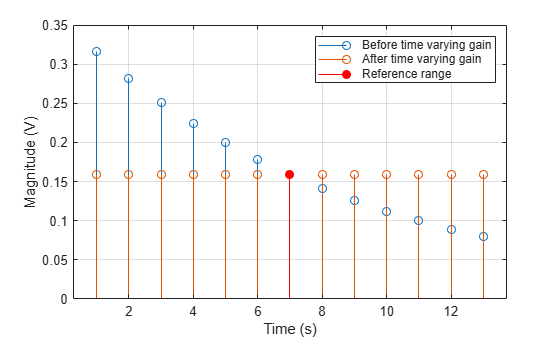Main Content

# phased.TimeVaryingGain

Time varying gain control

## Description

The `TimeVaryingGain` object applies a time varying gain to input signals. Time varying gain (TVG) is sometimes called automatic gain control (AGC).

To apply the time varying gain to the signal:

1. Define and set up your time varying gain controller. See Construction.

2. Call `step` to apply the time varying gain according to the properties of `phased.TimeVaryingGain`. The behavior of `step` is specific to each object in the toolbox.

Note

Starting in R2016b, instead of using the `step` method to perform the operation defined by the System object™, you can call the object with arguments, as if it were a function. For example, ```y = step(obj,x)``` and `y = obj(x)` perform equivalent operations.

## Construction

`H = phased.TimeVaryingGain` creates a time varying gain control System object, `H`. The object applies a time varying gain to the input signal to compensate for the signal power loss due to the range.

`H = phased.TimeVaryingGain(Name,Value)` creates an object, `H`, with each specified property Name set to the specified Value. You can specify additional name-value pair arguments in any order as (`Name1`,`Value1`,...,`NameN`,`ValueN`).

## Properties

 `RangeLossSource` Source of range losses Specify the source of range losses as either `'Property'` or ```'Input port'```. When you specify `RangeLossSource` as `'Property'`, the range loss for each sample is set in the `RangeLoss` property. When you specify the `RangeLossSource` as ```'Input port'```, the range losses are specified using an input argument to the `step` method. Default: `'Property'` `RangeLoss` Loss at each input sample range Specify the loss due to range as a vector — elements correspond to the samples in the input signal. Units are in dB. This property can have single or double precision. Default: `0` `ReferenceLoss` Loss at reference range Specify the loss at a given reference range as a scalar. Units are in dB. This property can have single or double precision. Default: `0`

## Methods

 step Apply time varying gains to input signal
Common to All System Objects
`release`

Allow System object property value changes

## Examples

collapse all

Apply time varying gain to a signal to compensate for signal power loss due to range.

First, create a signal with range loss. Set the reference loss to 16 dB.

```rngloss = 10:22; refloss = 16; t = (1:length(rngloss))'; x = 1./db2mag(rngloss(:));```

Then add gain to compensate for range loss.

```gain = phased.TimeVaryingGain('RangeLoss',rngloss,'ReferenceLoss',refloss); y = gain(x);```

Plot the signal with loss and the compensated signal.

```tref = find(rngloss==refloss); stem([t t],[abs(x) abs(y)]) hold on stem(tref,x(tref),'filled','r') xlabel('Time (s)'); ylabel('Magnitude (V)') grid on legend('Before time varying gain','After time varying gain',... 'Reference range')```expand all

## References

 Edde, B. Radar: Principles, Technology, Applications. Englewood Cliffs, NJ: Prentice Hall, 1993.

 Skolnik, M. Introduction to Radar Systems, 3rd Ed. New York: McGraw-Hill, 2001.

## See Also

Introduced in R2011a

## Support

#### Exploring Hybrid Beamforming Architectures for 5G Systems

Download white paper